# Power Query Formula Language M : Table Functions Part 1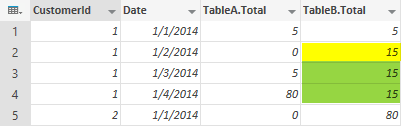In previous post you’ve learned about Formula language of the Power Query known as "M". you’ve learned that M is a functional language that apply data transformations through script. In examples of previous post you’ve learned some of table functions such as Table.AddColumn, Table.Sort, Table.Join, and Table.PrefixColumns. In this post we will discover more table functions through an example.

In this post you will learn these functions:

Table.RemoveColumns

Table.ReorderColumns

Table.SelectColumns

Table.Sort

Table.ReplaceValue

Table.FillDown

Table.RenameColumns

This function renames one or more columns of a table to desired name(s).

let’s apply this function on a sample table. here is the sample table generated from previous example: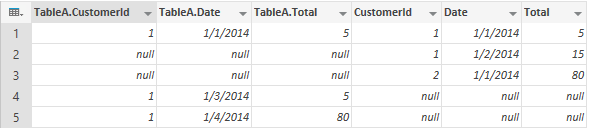this is the script that generates above table:

let
TableA = #table({"CustomerId", "TranDate","TranCount"},
{
{1,DateTime.FromText("2014-01-01 01:00:00.000"),10},
{1,DateTime.FromText("2014-01-01 02:00:00.000"),5},
{1,DateTime.FromText("2014-01-03 01:00:00.000"),5},
{1,DateTime.FromText("2014-01-04 02:00:00.000"),80}
}),

TableB = #table({"CustomerId", "TranDate","TranCount"},
{
{1,DateTime.FromText("2014-01-01 02:00:00.000"),10},
{1,DateTime.FromText("2014-01-01 03:00:00.000"),5},
{1,DateTime.FromText("2014-01-02 01:00:00.000"),20},
{1,DateTime.FromText("2014-01-02 03:00:00.000"),15},
{2,DateTime.FromText("2014-01-01 01:00:00.000"),5},
{2,DateTime.FromText("2014-01-01 02:00:00.000"),80}
}),
TableATransformed=Table.Sort(
,{"CustomerId","TranDate"}
) ,
TableBTransformed=Table.Sort(
,{"CustomerId","TranDate"}
)  ,
TableAGrouped=Table.Group(TableATransformed,{"CustomerId","Date"},{"Total",each List.Last([TranCount])}),
TableBGrouped=Table.Group(TableBTransformed,{"CustomerId","Date"},{"Total",each List.Last([TranCount])}),
ResultTable=Table.Join(Table.PrefixColumns(TableAGrouped,"TableA"),{"TableA.CustomerId","TableA.Date"},TableBGrouped,{"CustomerId","Date"},JoinKind.FullOuter)
in
ResultTable

Now we want to rename last three column of the table with a new prefix "TableB.". Here is how we can do that with Table.RenameColumns

let

…..

,RenamedTable=Table.RenameColumns(ResultTable,{ {"CustomerId","TableB.CustomerId"},{"Date","TableB.Date"},{"Total","TableB.Total"} })

in
RenamedTable

Result would be the below table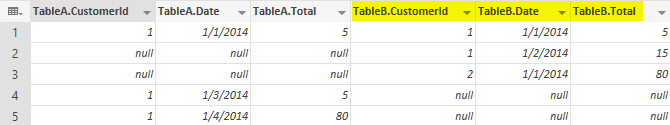Here is the structure of Table.RenameColumns

Table.RenameColumns(<table>,( {<column name before change>,<column name after change>},{repeat for more columns} } )

This function adds a column to the table. You would assign name of the new column and the script to generate new column. the new column generator can be a static value or an expression.

In this example we want to add three columns that be generated based on expressions from existing columns of RenamedTable. we want a new CustomerId Column to be generated from TableA.CustomerId, but if it is null, then it should get its value from TableB.CustomerId.

Here is the script to generate the new CustomerId Column:

in

Result table showed below: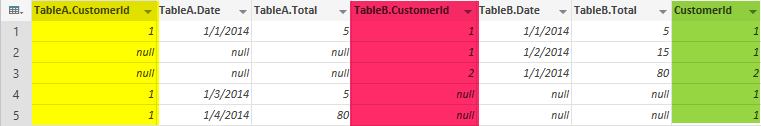As you see in the above expression we used IF structure which is the very handy conditional structure in M. the <IF> structure is simply as below:

If <condition>

then <if true>

else <if false>

The good thing about If is that it can be used within expressions to build the table/record/lists and etc (as you seen in this example).

The EACH keyword is required to generate expression for each record of the dataset.

here is structure of Table.AddColumn function:

Table.AddColumn(<table>,<new column name>,<new column generator>,<data type optional>)

in

Result showed below: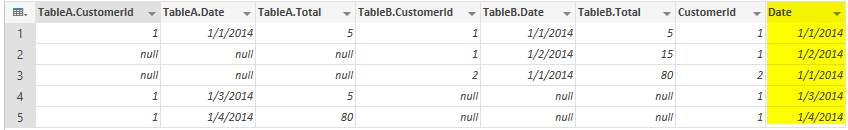Table.RemoveColumns

This function removes one or more columns from the table.

we can use this function to remove extra columns such as TableA.CustomerId, TableB.CustomerId, TableA.Date, and TableB.Date from the table.

Here is the script:

in
ColumnsRemoved

Result would be as below:structure of Table.RemoveColumns is as below:

Table.RemoveColumn(<table>,{columns to remove separated by comma})

Table.ReorderColumns

changes order of columns in table.

here is the expression that we used to reorder columns of this table:

,ColumnsOrdered=Table.ReorderColumns(ColumnsRemoved,{"CustomerId","Date","TableA.Total","TableB.Total"})
in
ColumnsOrdered

Result would be as below: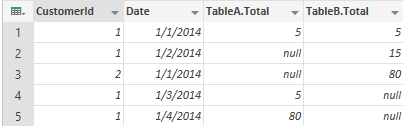here is the structure of Table.ReorderColumns

Table.ReorderColumns(<table>,{order of columns as desired in output})

Table.SelectColumns

This function selects specific columns from a table with the order defined. This function is a combination of Table.RemoveColumns and Table.ReorderColumns.

this script will result same table as above but with only a single function Table.SelectColumns instead of using Table.RemoveColumns and Table.ReorderColumns.

in
ColumnsSelected

Result would be the sameTable.Sort

Sorts a table on the desired ordering list

,SortedTable=Table.Sort(ColumnsSelected,{"CustomerId","Date"})
in
SortedTable

Result would be as below: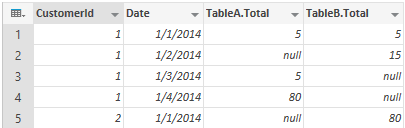Here is the Table.Sort function structure

Table.Sort(<table>,{sorting criteria})

Table.ReplaceValue

This function replace all old values with new value from the specific column in the table.

the expression below would replace all NULL values in TableA.Total with 0.

,ValueReplaced=Table.ReplaceValue(SortedTable,null,0,Replacer.ReplaceValue,{"TableA.Total"})
in
ValueReplaced

Result showed below: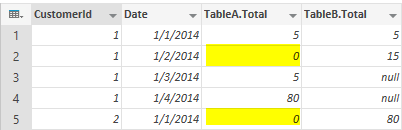Here is the structure of Table.ReplaceValue function

Table.ReplaceValue(<table>,<old value>,<new value>,<replacer function>,{column name})

replacer function can be Replacer.ReplaceValue or Replacer.ReplaceText

Table.FillDown

This function will fill the value from upper record down to the null values of the records after that.

Here is the example: (we want to fill down the values of columns TableB.Total, that means if a record has null value for this column, then the value would be fetched from the most recent top record that has a not null value)

,FilledDown=Table.FillDown(ValueReplaced,"TableB.Total")
in
FilledDown

result would be as below:Here is the structure of Table.FillDown function

Table.FillDown(<table>,<Column>)

This function adds an identity auto increment column to the table. you can specify the column name, initial value, and the increment seed.

This is a sample expression:

in

Result is as below: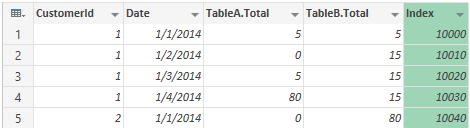Here is the structure for this function:

Here is the full script for this example:

let
TableA = #table({"CustomerId", "TranDate","TranCount"},
{
{1,DateTime.FromText("2014-01-01 01:00:00.000"),10},
{1,DateTime.FromText("2014-01-01 02:00:00.000"),5},
{1,DateTime.FromText("2014-01-03 01:00:00.000"),5},
{1,DateTime.FromText("2014-01-04 02:00:00.000"),80}
}),

TableB = #table({"CustomerId", "TranDate","TranCount"},
{
{1,DateTime.FromText("2014-01-01 02:00:00.000"),10},
{1,DateTime.FromText("2014-01-01 03:00:00.000"),5},
{1,DateTime.FromText("2014-01-02 01:00:00.000"),20},
{1,DateTime.FromText("2014-01-02 03:00:00.000"),15},
{2,DateTime.FromText("2014-01-01 01:00:00.000"),5},
{2,DateTime.FromText("2014-01-01 02:00:00.000"),80}
}),
TableATransformed=Table.Sort(
,{"CustomerId","TranDate"}
) ,
TableBTransformed=Table.Sort(
,{"CustomerId","TranDate"}
)  ,
TableAGrouped=Table.Group(TableATransformed,{"CustomerId","Date"},{"Total",each List.Last([TranCount])}),
TableBGrouped=Table.Group(TableBTransformed,{"CustomerId","Date"},{"Total",each List.Last([TranCount])}),
ResultTable=Table.Join(Table.PrefixColumns(TableAGrouped,"TableA"),{"TableA.CustomerId","TableA.Date"},TableBGrouped,{"CustomerId","Date"},JoinKind.FullOuter)
,RenamedTable=Table.RenameColumns(ResultTable,{ {"CustomerId","TableB.CustomerId"},{"Date","TableB.Date"},{"Total","TableB.Total"} })
,ColumnsOrdered=Table.ReorderColumns(ColumnsRemoved,{"CustomerId","Date","TableA.Total","TableB.Total"})
,SortedTable=Table.Sort(ColumnsSelected,{"CustomerId","Date"})
,ValueReplaced=Table.ReplaceValue(SortedTable,null,0,Replacer.ReplaceValue,{"TableA.Total"})
,FilledDown=Table.FillDown(ValueReplaced,"TableB.Total")